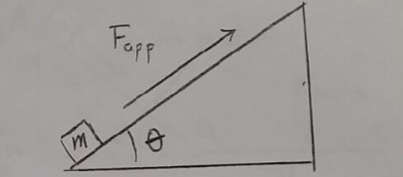# Problem: Consider the arrangement shown in the figure. A box of mass m = 5.00 kg is pushed up a slope of inclination angle θ = 25° by an applied force Fapp. The coefficients of static and kinetic friction between the box and the inclined plane are μs = 0.30 and μk = 0.20 respectively. Suppose the applied force has magnitude Fapp = F1 = 26.0 N. With the help of your diagram, determine the static frictional force acting on the box, assuming that the given applied force is not enough to start the box moving.

###### Problem Details

Consider the arrangement shown in the figure. A box of mass m = 5.00 kg is pushed up a slope of inclination angle θ = 25° by an applied force Fapp. The coefficients of static and kinetic friction between the box and the inclined plane are μs = 0.30 and μk = 0.20 respectively. Suppose the applied force has magnitude Fapp = F1 = 26.0 N. With the help of your diagram, determine the static frictional force acting on the box, assuming that the given applied force is not enough to start the box moving.